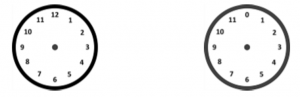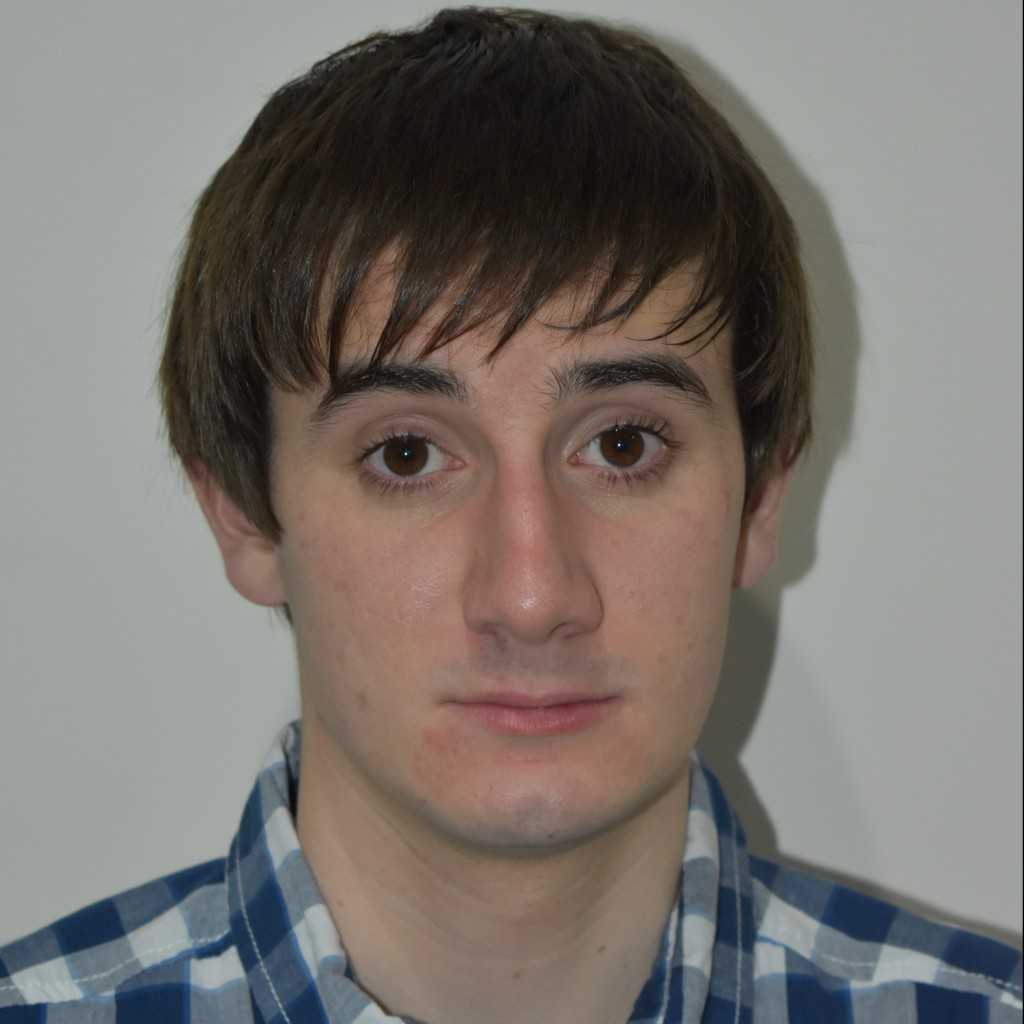Group theory is an important topic in pure mathematics that has many different applications. Examples of mathematical groups are all around us. This blog post looks at an example of an everyday object (a clock) and looks at how this works as a group. We look at the properties required for this to be a group and look at the additional properties that this group has.

Consider adding numbers together on a clock. Four plus three is seven, as is usual, but adding ten and five gives three, because the clock loops around. Suppose we change the clock so that it starts at zero instead of twelve. Now the clock goes from zero to eleven, so eight plus four now equals zero if we do addition on this clock. Mathematicians call this a group. A group consists of a set, which is our numbers from zero to twelve, and a binary operation. A binary operation takes two things and turns it into one thing. Addition is our binary operation, because it takes two numbers and turns them into one number. Multiplication, division, and subtraction are all binary operations.There are some properties required for something to be a group. The binary operation must not take things outside the set. If we weren’t doing addition on a clock, ten plus four would equal fourteen which isn’t in our set of numbers. Another requirement is that the group has something called an identity element. This is something that if you take a binary operation of this and something else, it doesn’t change the other thing. In our example, this is zero. Adding zero to something doesn’t change it. For any number in our set, we can always find another number if you add them together you get zero. For example, we can add nine to three to get zero. Everything in a group must be able to be turned into the identity. The final property is associativity, which says that adding brackets to an equation doesn’t change it. Two plus five plus three is the same if you add two and five first or add five and three first.

There are some other properties that this group specifically has which other groups don’t have. If we start with only the number ‘one’, we can add one to itself repeatedly and get all the numbers in our set (Keep in mind that eleven plus one is zero on our clock). Mathematicians call one a generator for this group since we can generate all the numbers in our set from just that number. If we started with the number ‘two’, we couldn’t generate any odd numbers from adding two to itself repeatedly. If we can find a generator for a group, then we call it a “cyclic” group. Our group only has 12 numbers in its set, so we call it a finite group as opposed to groups with infinite things in their set. Finally, addition is the same if you swap the numbers that you’re adding with each other. Five plus two and two plus five are the same. We call this commutative. Subtraction is not commutative, five subtract two is not the same as two subtract five. We would call the group we have a finite, cyclic, abelian (commutative) group. My research project mainly involved finite cyclic groups.

Aaron Jenkins
University of Western AustraliaView Aaron Jenkins
Profile